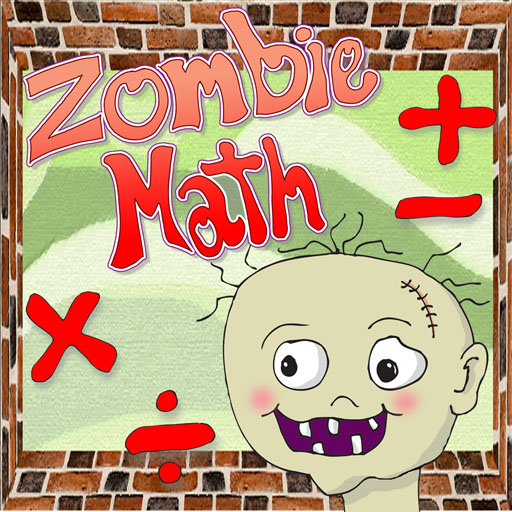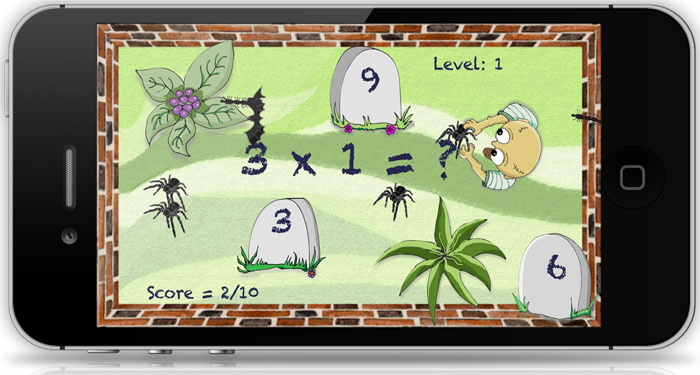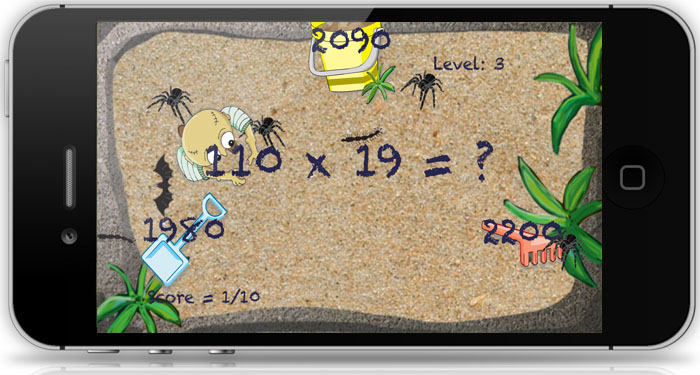A Friendly Zombie Helps Kids Learn Math...A Fun Game for Elementary Students to Practice Math SkillsCheck out our new Reading Series Series for Elementary age Students! Zombie Math provides practice in addition, subtraction, multiplication, and division. Three levels of problems are presented during the game. The levels increase in difficulty. Within each of the levels problems are randomly generated so every game is a unique experience. The game begins after the player selects a math skill and number of problems (10, 25 or 50). This app is appropriate for elementary age students who are in grades 3 through 6 or older students who need more practice to improve math skills. [ See also Math Bingo K-6 ]Select the Level and Number of Problems for Each Game Tap the settings icon and use a slider to select an appropriate level for the game. Once the skill level has been selected the players tap the 'GO' button to indicate that they are ready to begin. Randomly generated math problems flash on the screen at the selected level. The student directs their zombie toward the correct answer by tapping the screen. Play continues until the student completes the specified number of problems for each level. This game is challenging and fun. The game provides fast action as students tap the screen to lead their zombie to the correct answer to randomly generated math problems.Level 1: Graveyard Scene At this level addition problems are basic facts where both addends are between 0 and 10. Similarly for subtraction, the subtrahend up to 20 and the minuend between 0 and 10. For multiplication, practice problems range from multiples of 0 to 10. Likewise, in division practice, the dividend is less than 20 and the divisor between 1 and 10.Level 2: Basketball Court At this level addition problems are basic facts where both addends are between 10 and 20. Similarly for subtraction, the subtrahend up to 40 and the minuend between 10 and 20. For multiplication, practice problems range from multiples of 5 to 15. Likewise, in division practice, the dividend is less than 400 and the divisor between 5 and 15.Level 3: Sandbox At this level addition problems are basic facts where both addends are between 20 and 40. Similarly for subtraction, the subtrahend up to 80 and the minuend between 20 and 40. For multiplication, practice problems range from multiples of 50 to 150 and a multiple of 10. Likewise, in division practice, the dividend is less than 200 and the divisor between 5 and 15.\$0.99